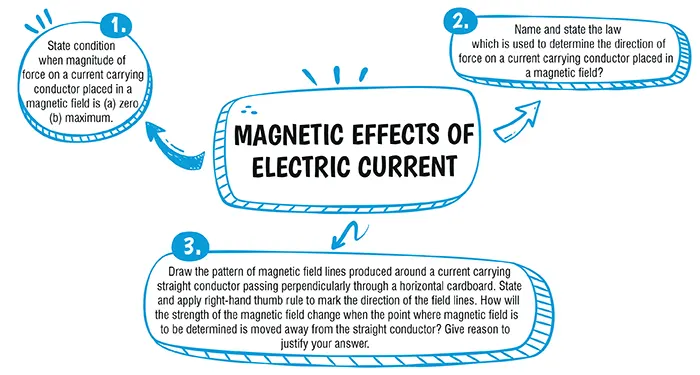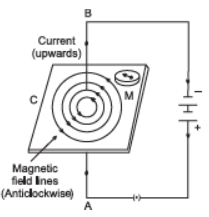Magnetic Effects of Electric Current

# Class 10 Science Chapter 12 Magnetic Effects of Electric Current Important QuestionsHere are some critical Chapter 12 Magnetic Effects of Electric Current Problems for Class 10 Science. These inquiries are intended to aid students in studying for and performing well on the CBSE Class 10 Science Examination 2023–24. Students can clear up their concerns and be ready for the exams by practising different types of questions. By answering these questions, you'll increase your confidence while also sharpening your problem-solving abilities

## Introduction

In Chapter 12 Magnetic Effects of Electric Current you will study about Magnetic Field and Field Lines, Magnetic Field due to a Current Carrying Conductor, Force on a Current carrying Conductor in a Magnetic Field, Electric Motor, Electromagnetic Induction, Electric Generator and Domestic Electric Circuits.### Class 10 Magnetic Effects of Electric Current Important Questions and Answers

#### (a) 2f (b) $$\frac{F}{2}$$(c) $$\frac{2 F}{4}$$(d) 4f

Ans. (d)

Explanation:
If the current is doubled in each wire the force will be 4F. This can be explained as follows:We know that the force of repulsion per unit length between two wires carrying current in opposite direction is:
$$\frac{F}{l}=\frac{\mu_0i_1i_2}{2\pi d}$$
Thus when both i1 and i2 are doubled, the force between them becomes four times.

#### (a) 4.22 × 10-7 T(b) 42.24 × 10-7 T(c) 422.4 × 10-7 T(d) 422.2 × 10-7 T

Ans. (d)

Explanation:
B = µ0ni
Where,
$$n=\frac{N}{L}$$
=$$\frac{4\pi×10^{-7}×210×8}{0.50}$$
= 422.2 × 10-5 T​

#### Q3. State condition when magnitude of force on a current carrying conductor placed in a magnetic field is (a) zero (b) maximum.

Explanation:
No force acts on a current carrying conductor when the current in the conductor is parallel to the magnetic field.
Maximum force is exerted on the current carrying conductor if it is perpendicular to the direction of magnetic field.

#### Q4. Name and state the law which is used to determine the direction of force on a current carrying conductor placed in a magnetic field?

Explanation:
The direction of force on a current carrying conductor placed in a magnetic field is obtained by the Fleming’s left hand rule.
This rule states that “ When forefinger, central finger and thumb of left hand are stretched in such a way that they are mutually perpendicular to each other, if the forefinger indicates direction of magnetic field, central finger indicates the direction of current, then thumb will indicate the direction of motion of conductor i.e., force on the conductor.

#### Q5. Draw the pattern of magnetic field lines produced around a current carrying straight conductor passing perpendicularly through a horizontal cardboard. State and apply the right-hand thumb rule to mark the direction of the field lines. How will the strength of the magnetic field change when the point where the magnetic field is to be determined is moved away from the straight conductor? Give reason to justify your answer.

Explanation:
Maxwell’s Right Hand Thumb rule states that if current carrying wire is imagined to be held in the right hand so that the thumb points in the direction of current, then the direction in which fingers encircle the wire will give the direction of magnetic field lines around the wire. If we hold the current carrying straight wire so that thumbs in upward direction points the direction of current, the direction of
magnetic field lines will be anticlockwise. The strength of the magnetic field is inversely proportional to the distance of the point of observation from the wire. So, as we move away from the wire the strength of the magnet decreases.#### CBSE Class 10 Science Chapter wise Important Questions

Chapter No. Chapter Name
Chapter 1 Chemical Reactions and Equations
Chapter 2 Acid, Bases and Salts
Chapter 3 Metals and Non-Metals
Chapter 4 Carbon and its Compounds
Chapter 5 Life Processes
Chapter 6 Control and Coordination
Chapter 7 How do Organisms Reproduce
Chapter 8 Heredity
Chapter 9 Light : Reflection and Refraction
Chapter 10 The Human Eye and the Colourful world
Chapter 11 Electricity
Chapter 12 Magnetic Effects of Electric Current
Chapter 13 Our Environment

#### Conclusion

oswal.io offers a thorough set of questions for learning the topic in a better way if you're looking to further practise and improve your grasp of the concepts covered in the chapter.

#### Q1 : What is meant by magnetic field?

Ans: Magnetic field : It is defined as the space surrounding the magnet in which magnetic force can be experienced.

#### Q2: A compass needle is placed near a current carrying straight conductor. State your observation for the following cases and give reasons for the same in each case.(a) Magnitude of electric current is increased.(b) The compass needle is displaced away from the conductor.

Ans:  (a) As the amount of magnetic field strength is directly proportional to the amount of current, so the deflection of the compass needle increases.
(b) Since magnetic field strength at a point is inversely proportional to the distance from the wire. Hence deflection of the compass decreases when it is displaced away from the conductor.

#### Q3 : Give reason for the following(i) There is either a convergence or a divergence of magnetic field lines near the ends of a current carrying straight solenoid.(ii) The current carrying solenoid when suspended freely rests along a particular direction.

Ans: (i) There is either a convergence or a divergence of magnetic field lines near the ends of a current carrying straight solenoid because it behaves similar to that of a bar magnet and has a magnetic field line pattern similar to that of a bar magnet. Thus the ends of the straight solenoid behave like poles of the magnet, where the converging end is the south pole and the diverging end is the north pole.
(ii) The current carrying solenoid behaves similar to that of a bar magnet and when freely suspended aligns itself in the north-south direction.

#### Q4 : Write one application of Fleming's left hand rule.

Ans: Fleming's left hand rule is used to find the direction of force on a current carrying conductor placed in a magnetic field acting perpendicular to the direction of current.

#### Q5 : State whether an alpha particle will experience any force in a magnetic field if (alpha particles are positively charged particles)(i) it is placed in the field at rest.(ii) it moves in the magnetic field parallel to field lines.(iii) it moves in the magnetic field perpendicular to field lines.Justify your answer in each case.

Ans: (i) No, an alpha particle will not experience any force if it is at rest, because only moving charge particles can experience force when placed in a magnetic field.
(ii) No, an alpha particle will not experience any force if it moves in the magnetic field parallel to field lines because a charged particle experiences force only when it moves at an angle other than 0° with magnetic field.
(iii) Alpha particles will experience a force in the direction perpendicular to the direction of magnetic field and direction of motion of alpha particle.

###### Copyright 2022 OSWAL PUBLISHERS Simplifying ExamsPhone:  (+91) 78959 87722
Mail: support@oswalpublishers.in